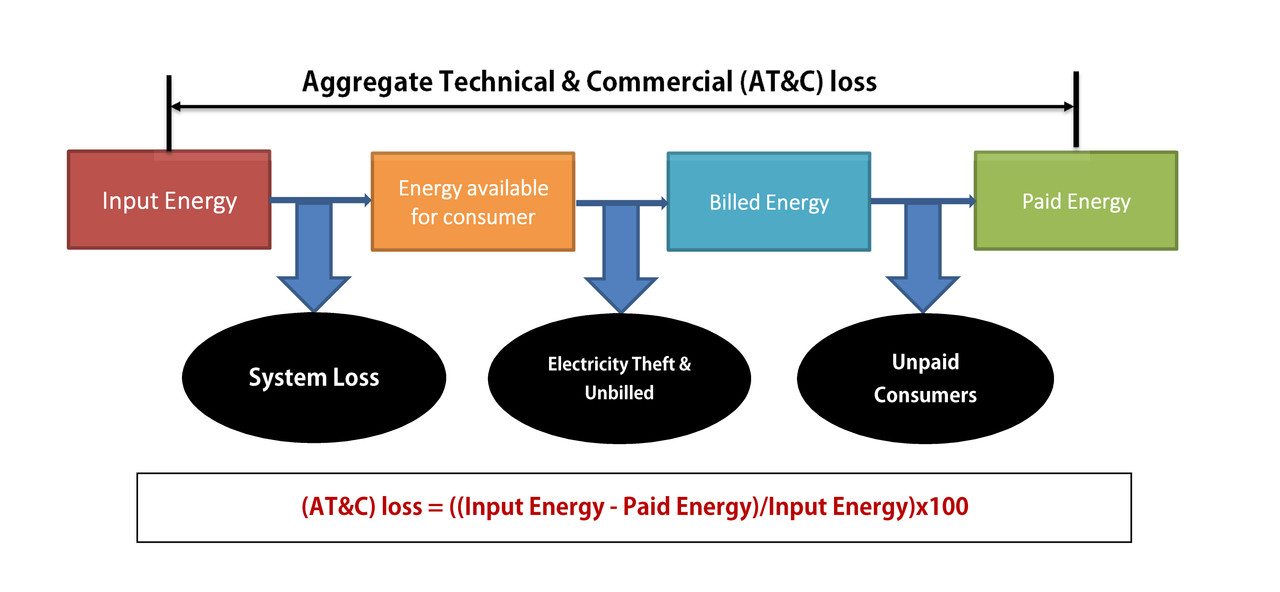#### What is Aggregate Technical & Commercial (AT&C) loss?### Aggregate Technical & Commercial (AT&C) loss

Aggregate Technical & Commercial (AT&C) loss is an actual measure of performance of a  power distribution system as it includes both technical losses and commercial losses. it shows the gap of input energy into the system and the units for which the payment is collected.

The aggregate technical and commercial losses shall be measured using the formula mentioned below:

• AT&C Losses = {1 - (Billing Efficiency X Collection Efficiency)} X 100
Where,
• Billing Efficiency = Billed Energy / Input Energy
• Collection Efficiency = Revenue Collected / Billed Amount (Current Assessment)### Billing Efficiency

Billing Efficiency is an indicator of the proportion of energy that has been billed (includes both metered and unmetered sales) to consumers in comparison to the energy supplied to an area.
• Billing Efficiency = Total Billed Unit (kWh) / Total Input Energy(kWh)

### Collection efficiency

Collection efficiency is an indicator of the proportion of an amount that has been collected from consumers w.r.t. amount billed to them. There are quite a few consumers who have a tendency to default on their payments for various reasons. Thus utility is not able to recover the entire amount billed by it, resulting in commercial losses.
• Collection Efficiency = Revenue Collected / Billed Amount

The aggregate technical and commercial losses shall also be measured using the average tariff rate (ATR) and through rate.
Where,

• Average Tariff Rate (ATR) is a simple mean of the applied rate of all categories.
• Through Rate is the average rate of revenue realized per unit of input energy.
• AT&C Loss = (1-Through Rate/ ATR)x100

How to calculate ATR?
ATR is nothing but ABR (Average billing rate) with 100% billing accuracy.
ABR = Current Assessment/Billed Energy.
one may calculate ABR of MU based consumers, result in ATR